## 在浏览器中进行深度学习：TensorFlow.js (十一）时间序列预测

• AR （自回归模型 Auto Regression）
• MA （移动平均 Moving Average ）
• ACF/PACF （自相关和偏自相关）
• ARIMA （AR和MA模型的结合）
• 卡尔曼滤波

### 数据导入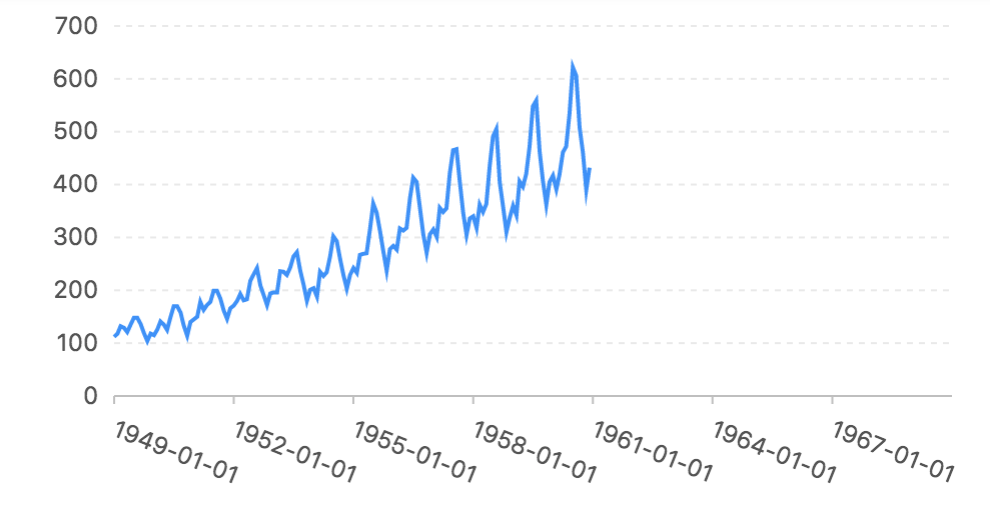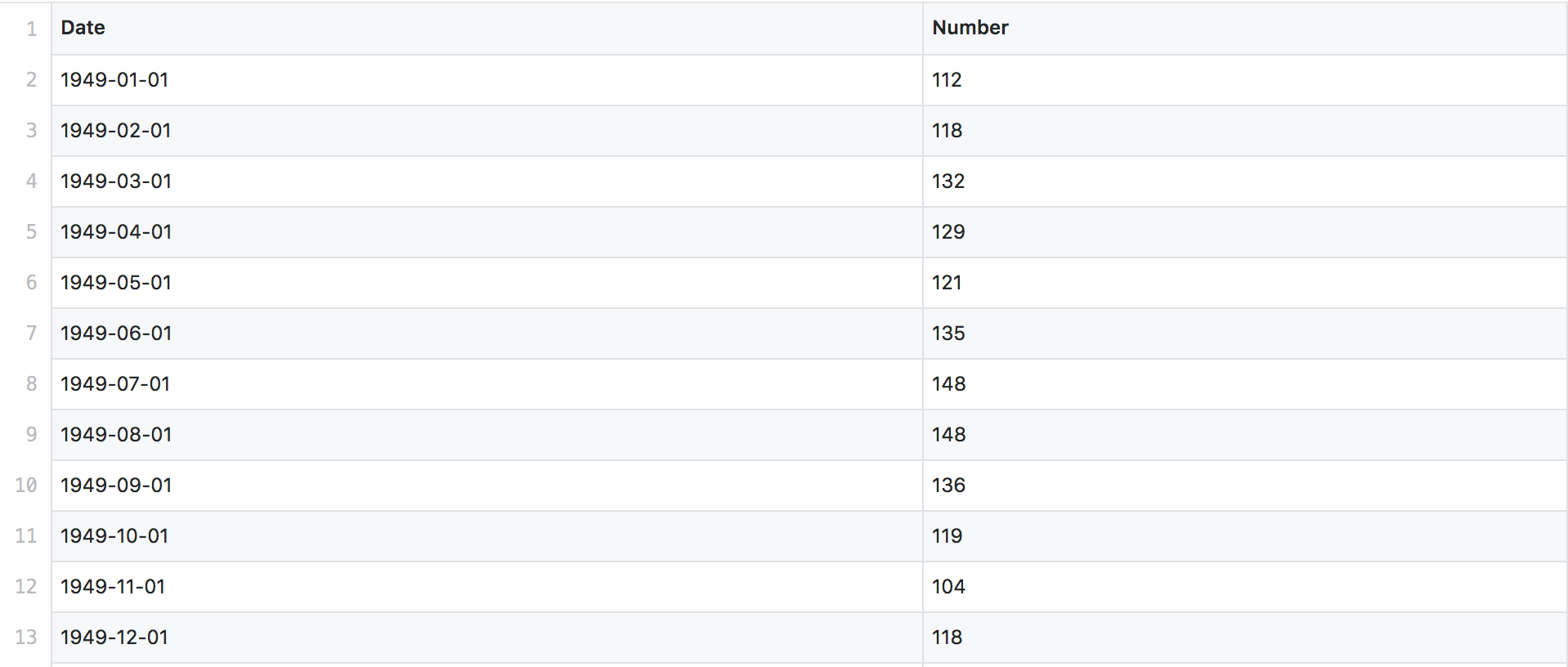``````async function loadData(path) {
return await d3.csv(path);
}

"https://cdn.jsdelivr.net/gh/gangtao/datasets@master/csv/air_passengers.csv"
);``````

### 数据准备

``````  // Normalize data with value change
let changeData = [];
for (let i = 1; i < airPassagnerData.length; i++) {
const item = {};
item.date = airPassagnerData[i].Date;
const val = parseInt(airPassagnerData[i].Number);
const val0 = parseInt(airPassagnerData[i - 1].Number);
item.value = val / val0 - 1;
changeData.push(item);
}``````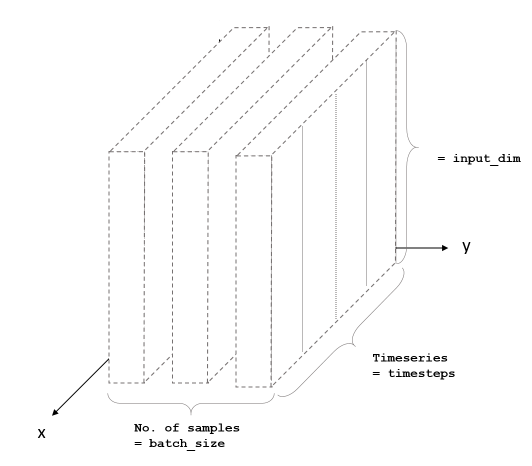LSTM需要一个三维的数据输入，分别对应Batch（数据是一批一批进入神经网络训练），Input是输入数据的size和Timestep，时间。所以我们在构造训练数据的时候，需要针对时间维度创建训练数据。

``````const STEP_SIZE = 3;
const STEP_NUM = 24;
const STEP_OFFSET = 1;
const TARGET_SIZE = 12;

function buildX(data, stepSize, stepNum, stepOffset) {
let xData = [];
for (let n = 0; n < stepNum; n += 1) {
const startIndex = n * stepOffset;
const endIndex = startIndex + stepSize;
const item = data.slice(startIndex, endIndex).map(obj => obj.value);
xData.push(item);
}
return xData;
}

function makeTrainData(data, stepSize, stepNum, stepOffset, targetSize) {
const trainData = { x: [], y: [] };

const length = data.length;

const xLength = stepSize + stepOffset * (stepNum - 1);
const yLength = targetSize;
const stopIndex = length - xLength - yLength;

for (let i = 0; i < stopIndex; i += 1) {
const x = data.slice(i, i + xLength);
const y = data.slice(i + xLength + 1, i + xLength + 1 + yLength);

const xData = buildX(x, stepSize, stepNum, stepOffset);
const yData = y;

trainData.x.push(xData.map(item => item));
trainData.y.push(yData.map(item => item.value));
}
return trainData;
}

const trainData = makeTrainData(
changeData,
STEP_SIZE,
STEP_NUM,
STEP_OFFSET,
TARGET_SIZE
);``````

• STEP_SIZE
这个是数据窗口的大小，3表示每一个数据包含三个值，数据input维度就是3
• STEP_NUM
这个是一共要走多少步，24表示走24步，那个Time维度就是24
• STEP_OFFSET
这个offset决定了时间窗口向前移动的时候，每次走多少个时间单位，我这里取1，也就是每次走一步，这样第一个数据和第二个数据其实存在两个重复值。
• TARGET_SIZE
这个是我要预测的时间长度，12表示预测12个月的数据。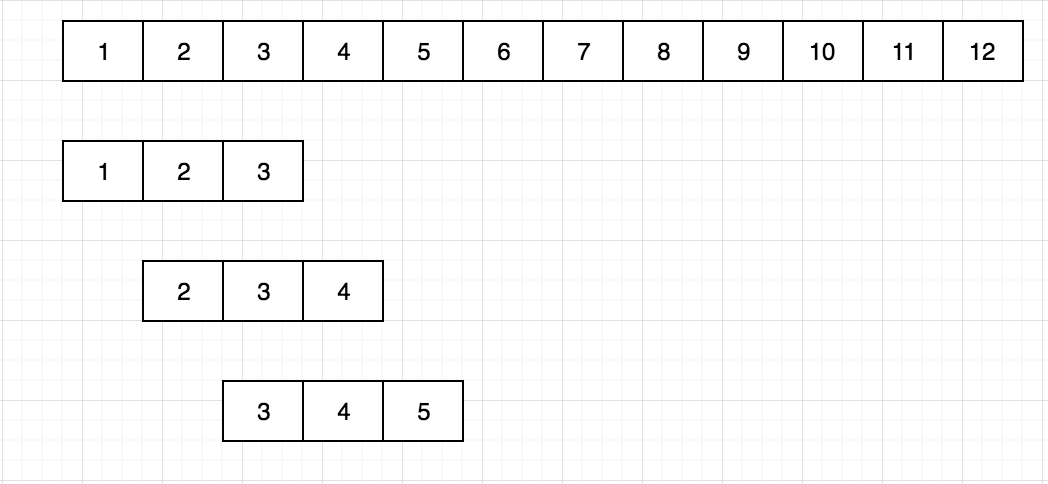### 模型构建

``````function buildModel() {
const model = tf.sequential();

//lstm input layer
const hidden1 = tf.layers.lstm({
units: LSTM_UNITS,
inputShape: [STEP_NUM, STEP_SIZE],
returnSequences: true
});

//2nd lstm layer
const output = tf.layers.lstm({
units: TARGET_SIZE,
returnSequences: false
});

tf.layers.dense({
units: TARGET_SIZE
})
);

//compile
const rmsprop = tf.train.rmsprop(0.005);
model.compile({
optimizer: rmsprop,
loss: tf.losses.meanSquaredError
});

return model;
}``````

``const saveResults = await model.save('downloads://model-name');``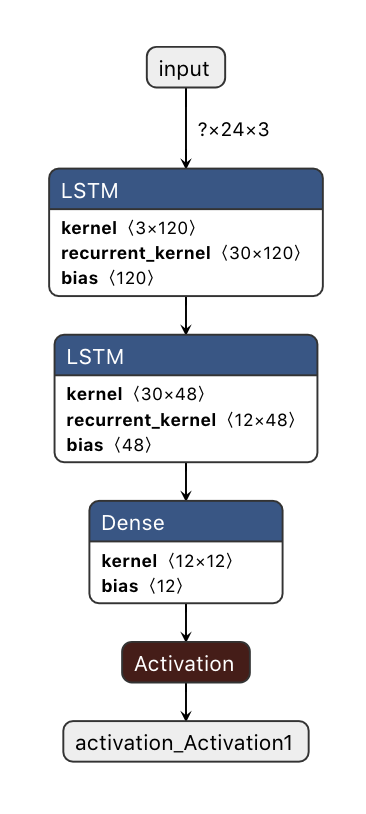### 模型训练

``````async function trainBatch(data, model) {
const metrics = ["loss", "val_loss", "acc", "val_acc"];
const container = {
name: "show.fitCallbacks",
tab: "Training",
styles: {
height: "1000px"
}
};
const callbacks = tfvis.show.fitCallbacks(container, metrics);

console.log("training start!");
tfvis.visor();

const epochs = config.epochs;
const results = [];
const xs = tf.tensor3d(data.x);
const ys = tf.tensor2d(data.y);

const history = await model.fit(xs, ys, {
batchSize: config.batchSize,
epochs: config.epochs,
validationSplit: 0.2,
callbacks: callbacks
});

console.log("training complete!");
return history;
}

const trainData = makeTrainData(
changeData,
STEP_SIZE,
STEP_NUM,
STEP_OFFSET,
TARGET_SIZE
);
const model = buildModel();
model.summary();``````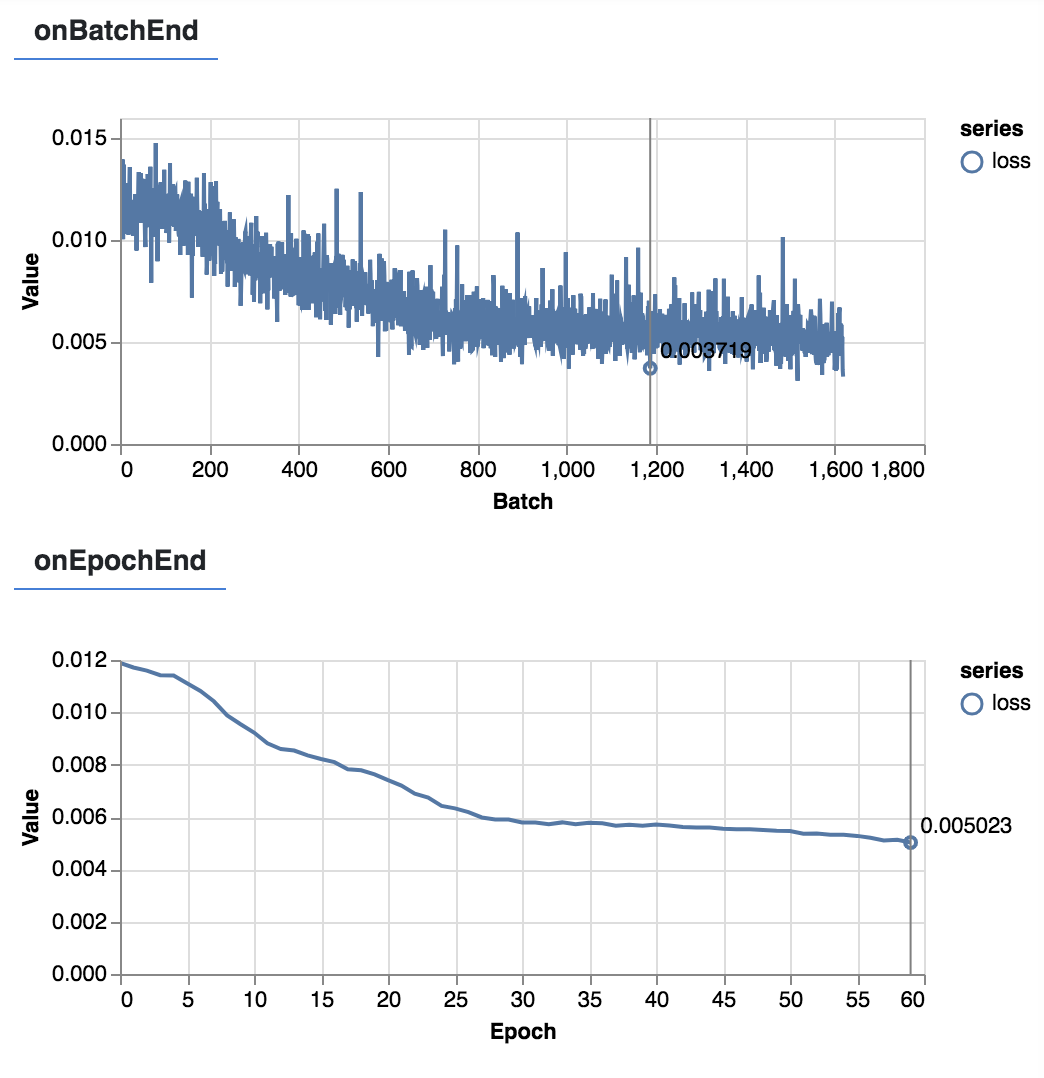### 预测

``````  async function predict(model, input) {
const prediction = await model.predict(tf.tensor([input])).data();
return prediction;
}

const inputStart = changeData.length - X_LEN;
const inputEnd = changeData.length;
const input = changeData.slice(inputStart, inputEnd);
const predictInput = buildX(input, STEP_SIZE, STEP_NUM, STEP_OFFSET);
const prediction = await predict(model, predictInput);

// re-constructe predicted value based on change
const base = airPassagnerData[airPassagnerData.length-1];
const baseDate = moment(new Date(base.Date));
const baseValue = parseInt(base.Number);

let predictionValue = [];
let val = baseValue;
for( let i = 0; i < prediction.length; i+=1) {
const item = {};
item.time = moment(date).format('YYYY-MM-DD');
item.value = val + val * prediction[i];
item.isPrediction = "Yes";
predictionValue.push(item);
val = item.value;
}

console.log(predictionValue);
let airPassagnerDataWithPrediction = [];
chartData.forEach(item => {
item.isPrediction = "No";
airPassagnerDataWithPrediction.push(item);
})
predictionValue.forEach(item => {
airPassagnerDataWithPrediction.push(item);
})``````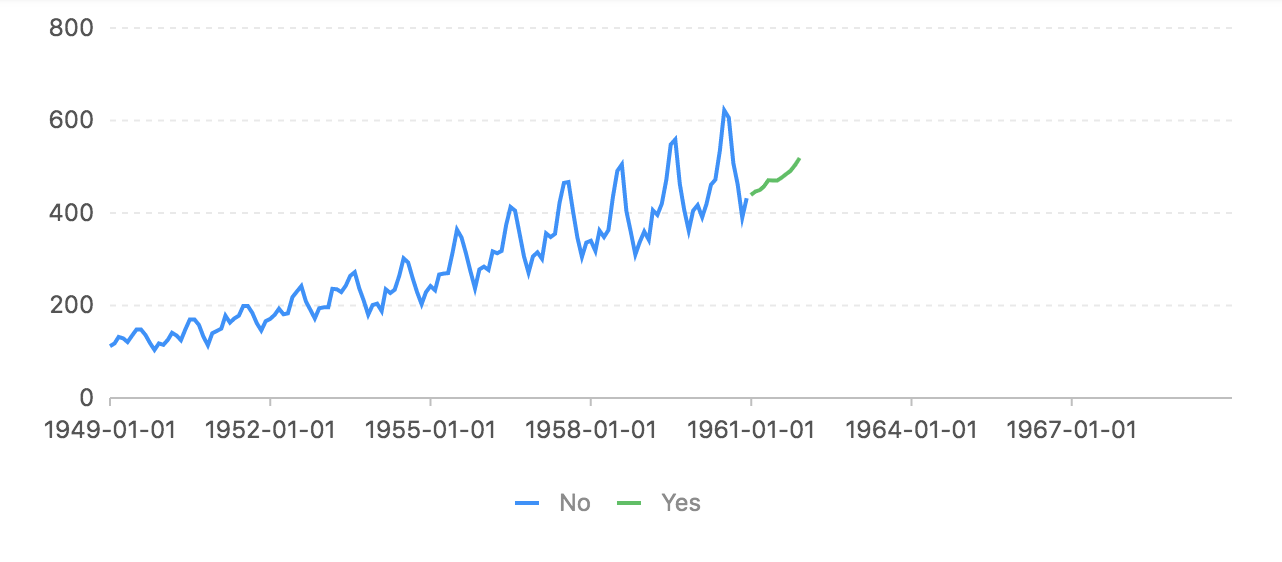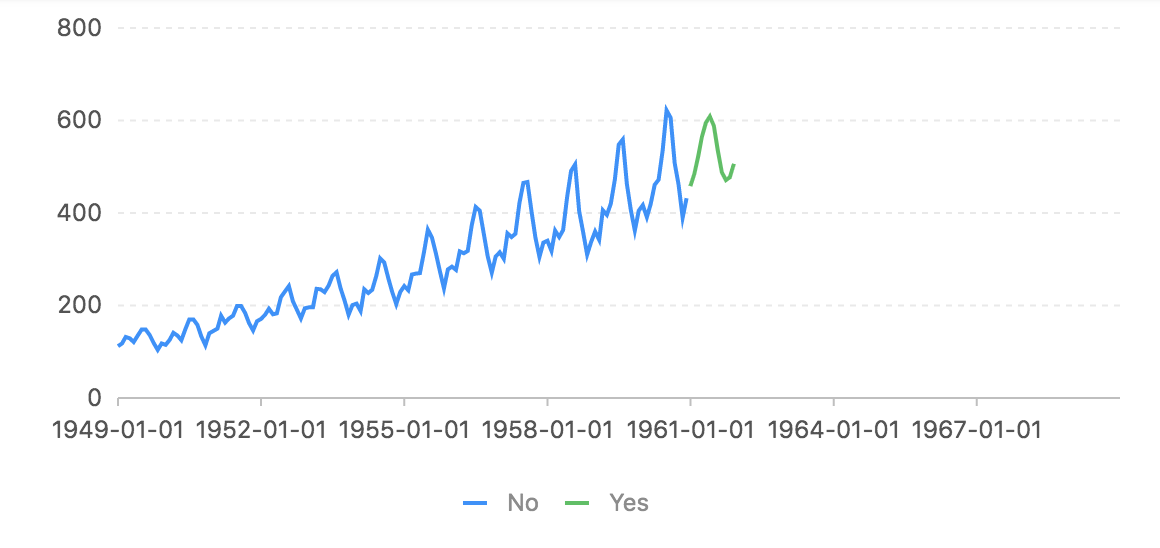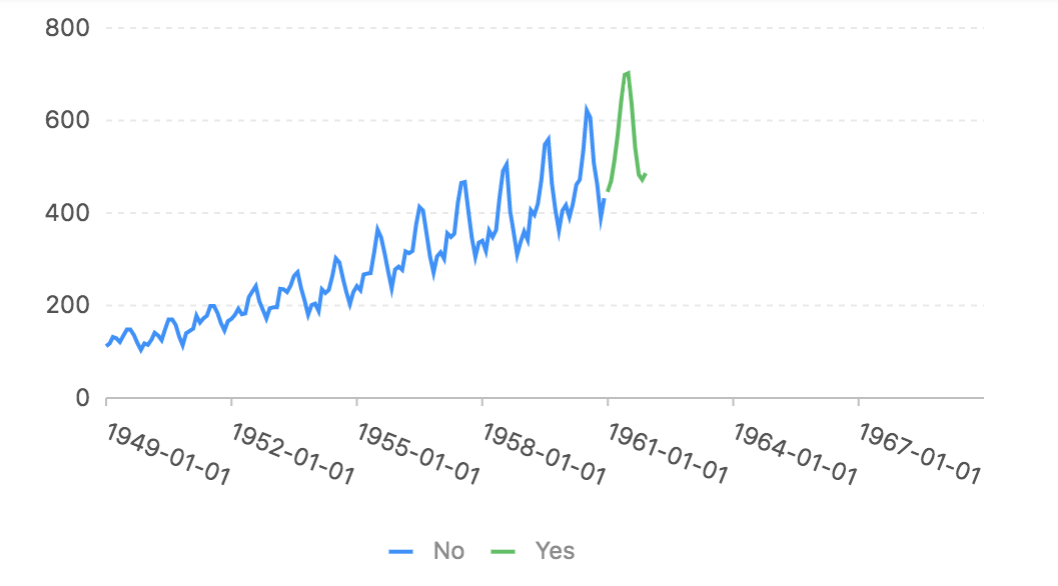### 总结

• 对于输入数据，一开始是的size是1，但是训练的效果很差，为了有好的训练效果，把Size增加到3，结果很不错。
• LSTM会有梯度消失的问题，如果选择合适的网络结构，参数需要有相当的经验和尝试。
• 在本文的问题中，复杂和更深的网络并不能带来效果的明显提升。
• 我们利用窗口滑动获取训练数据的过程和卷积操作很想，据说利用CNN也可以达到不错的效果，有兴趣的同学可以试一下。

### 参考

(c)注：本文转载自https://my.oschina.net/taogang/blog/3051709，转载目的在于传递更多信息，并不代表本网赞同其观点和对其真实性负责。如有侵权行为，请联系我们，我们会及时删除.Contact